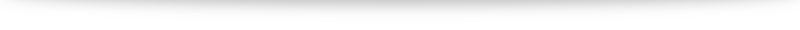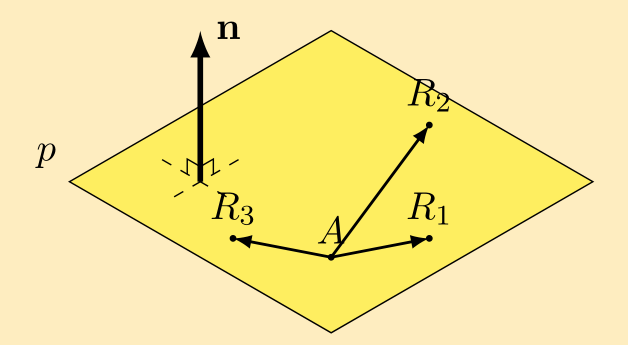# Alternative method to find food of perpendicular (without using vector projections)

In my video (link to be updated) I have worked out examples of how to use projection vectors to find the foot of perpendicular (for both lines and planes). The benefits of this method

1. it is faster,
2. it uses a similar concept for both lines and planes with slight modifications.

The downsides, however, is that it involves a rather unwieldy projection vector formula $(\mathbf{a}\cdot\mathbf{\hat{b}}) \mathbf{\hat{b}}$ and that minor details make a huge difference to whether we get the correct answer (e.g. where are our vectors pointing, $\overrightarrow{AB}$ or $\overrightarrow{BA}$? Is our final projection vector $\overrightarrow{AF}$ or $\overrightarrow{FB}$?).
In this post I will offer an alternative method to find foot of perpendicular using earlier concepts that some students find easier to understand.

### Finding foot of perpendicular to a line# Alternative method to find the line of intersection between two planes

In the video (link to be updated), we went through how to find the line of intersection between two planes using a GC. In this post, we will discuss an alternative method to accomplish this without the use of technology. Just like in our video, we will use the following example:
Find the equation of the line of intersection between $$p_1: \mathrm{r} \cdot \begin{pmatrix} 1 \\ 0 \\ 5 \end{pmatrix} = 7, \quad p_2: \mathrm{r} \cdot \begin{pmatrix} 1 \\ 1 \\ -1 \end{pmatrix} = -3.$$
The two important things we need to find an equation for a line are (1) a point on the line and (2) the direction vector parallel to the line. We will thus tackle the two separately:

#### Finding the direction vector

The key observation here is that the direction vector of the line of intersection is perpendicular to the normal vectors of both planes (try to visualize it!). Thus, to find the direction vector of the line of intersection, we can use the cross product. Hence# Why $\mathbf{r}\cdot\mathbf{n} = \mathbf{a}\cdot\mathbf{n}$ for planes?

When I was first learning about equations of planes, the vector form of a plane $\mathbf{r} = \mathbf{a} + \lambda \mathbf{d_1} + \mu \mathbf{d_2}$ made a lot more sense to me: pick different values of $\lambda$ and $\mu$, and we will end up at different points on the plane.

And while I can see why the vector form isn’t as useful for further applications because there are so many different direction vectors (infinite, in fact), and that the normal vector is a much better way to describe planes because it is unique (up to a scalar multiple), the equation $\mathbf{r}\cdot\mathbf{n} = \mathbf{a}\cdot\mathbf{n}$ just isn’t very intuitive and didn’t make a lot of sense. In today’s post I’d try my best to explain the origins of such an equation.

Understanding how the equation comes about boils down to knowing what $\mathbf{a}$ and $\mathbf{r}$ represent. $\mathbf{a}$ is the position vector of a fixed point $A$ that we already know lies on the plane. In forming an equation of an object, we will like to know what equation any other random points on the object satisfies. (For example, we say $y=2x+3$ is an equation of a line because we can check that $(0,3)$ satisfies the equation (and hence lies on the line) while $(1,4)$ does not). $\mathbf{r}$ is used to represent the position vector of a random point on our plane. We will call the point $R$.So, in the picture above, we have fixed $A$, and randomly pick a few points which we named $R_1, R_2$ and $R_3$. It turns out that, regardless of which $R$ we take, we can form a direction vector $\overrightarrow{AR}$. And the normal vector of our plane, $\mathbf{n}$ is so special in the sense that $\overrightarrow{AR}$ is perpendicular to $\mathbf{n}$. Thus we have $\overrightarrow{AR} \cdot \mathbf{n} = 0$.

The rest boils down to some algebra manipulation. $\overrightarrow{AR} = \overrightarrow{OR}-\overrightarrow{OA}$ so $(\mathbf{r}-\mathbf{a})\cdot \mathbf{n} = 0$. Expansion gives $\mathbf{r} \cdot \mathbf{n} – \mathbf{a} \cdot \mathbf{n} = 0$ which leads us to our familiar $\mathbf{r}\cdot\mathbf{n} = \mathbf{a}\cdot\mathbf{n}$.

Hopefully this proof and explanation helps, but I bet that even then the equation still looks a bit weird (it did to me years ago too!). The trick is to simply keep practicing with it and it’d become second nature in no time!# Proof of the dot product formula

## My experiences with the dot product

I struggled quite a bit when I first encountered the dot/scalar product in school. The dot product between two vectors $\mathbf{a}$ and $\mathbf{b}$ was defined to be $\mathbf{a} \cdot \mathbf{b} = |\mathbf{a}||\mathbf{b}| \cos \theta$ where $\theta$ is the angle between the two vectors. This definition posed a bit of stumbling block for me. Up until this point, most definitions has come to be pretty intuitive (and if not at first, it starts feeling reasonably natural usually within a week or two of working with it). This formula or definition seems like it is simply plucked out of midair.# Is $\sqrt{x^2} = x$? The many different modulus functions.

What is $\sqrt{x^2}$? Most of us will intuitive say "$x$": after all, $\sqrt{9} = \sqrt{3^2} = \sqrt{3}$, for example. However, what is $\sqrt{(-3)^2}$?

It is not $-3$ and is in fact $\sqrt{(-3)^2} = \sqrt{9} = 3$. Hence $\sqrt{x^2} =x$ is only valid if $x$ is non-negative. If $x$ is negative, it turns out that $\sqrt{x^2} = -x$.

The reason for this stems from definition: the symbol $\sqrt{ \cdot}$ is defined to be the "positive square root" when there are actually two possible square roots to every positive real number (this is the reason the equation $x^2 = k$ has two solutions, $\pm \sqrt{k}$, for positive $k$).

A compact way to summarize: